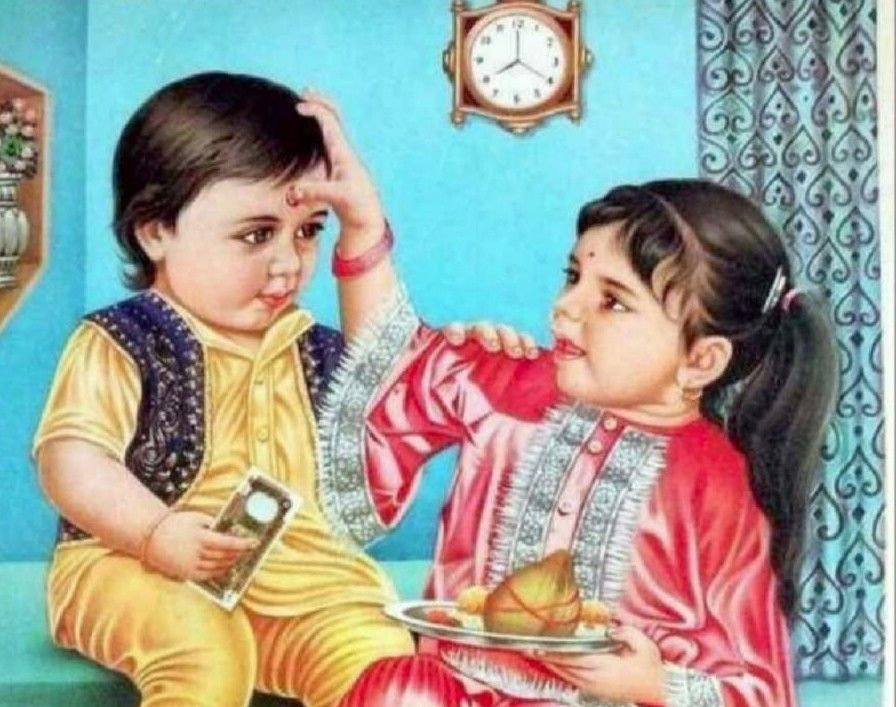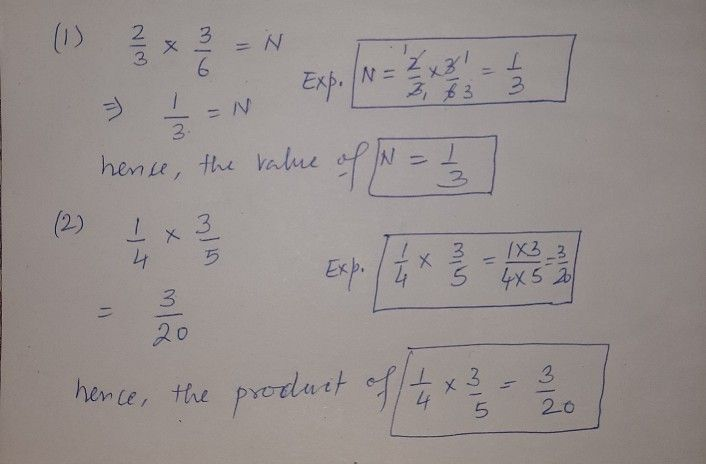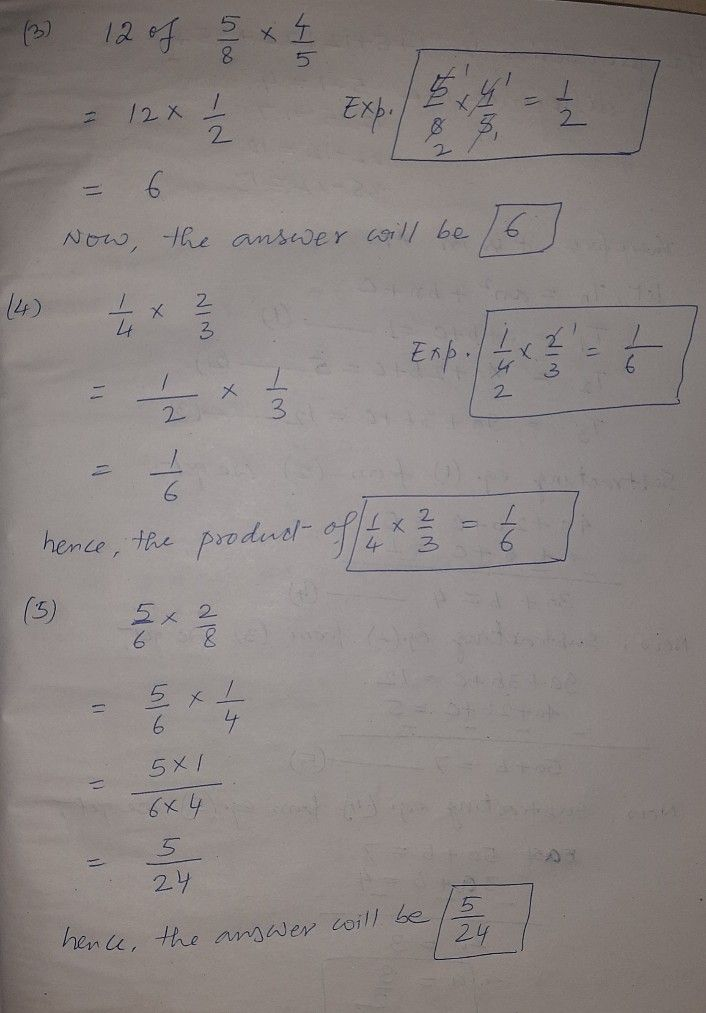Symbol
Problem$1\right)$ In the equation $x^{1}=N$ what is the value of N? $2\right)$ If you multiply $x$ what will be the product? $4$ $\dfrac {5} {n}\times -7$ $3\right)$ What is $12$ ofx? $4\right)$ What is the product of $1$ $4$ $x\dfrac {2} {3}$ ? $5\right)$ Multiply $1$ $-\times \dfrac {2} {0}$ What is the answer? Activity $3$
1st-6th grade
Other
Search count: 106
SolutionQanda teacher - AnishaStudent
Thank you